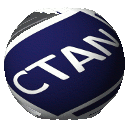# Directory `info/pstricks_calcnotes`

```This package presents three applications of PSTricks for making LaTeX lecture notes in
Calculus.

Application 1: Drawing approximations to the area under a graph by rectangles.
Application 2: Drawing the vector field of an ordinary differential equation of order one.
(The remarks on this application give a way of coloring arrows properly for a vector field.)
Application 3: Drawing partitions of a simply connected plane domain.
(There is a modified version of this application: AppThreePDF.pdf or AppThreePS.ps.)

Author: Le Phuong Quan